## A Demonstration of What Mathematica Can Do for High School Students and College Freshmen

Mathematica has a huge collection of functions and features in the user interface that isn't even hinted to in this demonstration. All that you see here are a few features high school students and college freshmen are likely to find useful.  The purpose here is to answer some questions about whether it is easy for them to use Mathematica for the some things they will likely need.

In the first example Mathematica finds all solutions of an equation.  As we see here Mathematica has no trouble with equations that involve variables.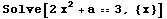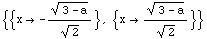In the next line Mathematica knows the exact value of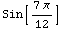.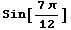If you want to approximate the last result you can use the next line.  Internally the result is computed to 16 decimal places, and there are ways to see as many digits as you want.  If however you triedyou would have got the approximate number because 7.0 is an approximate number.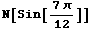0.9659258262890682`

Mathematica can easily perform algebra tasks like the one in the next line.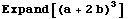Mathematica has powerful capabilities to simplify expressions. In the next line a non-trivial expression in trigonometric functions is simplified.-2+3 Cos[Theta]+3 Sin[Theta]

In the next line Mathematica figures out thatMathematica is also known for its amazing ability to perform complicated sums and integrals!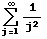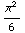Next we use Mathematica to make a plot of a polynomial.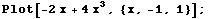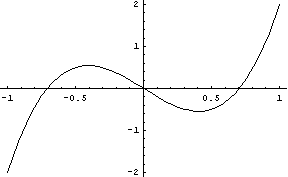Next we use Mathematica to make a 3D plot of a polynomial in variables (x,y).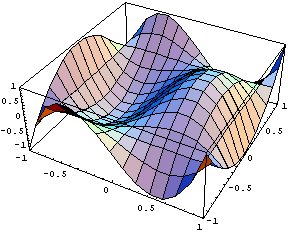You might prefer to get a graphic like the one below instead, and one can fix it so 3D plots always come out this way.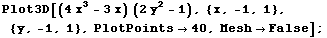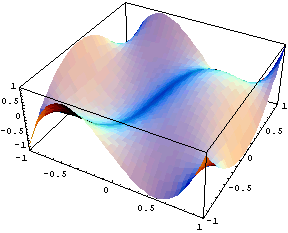Converted by Mathematica      January 25, 2000
Styles converted by a program by Reinhold Kainhofer ( reinhold@wolfram.com )